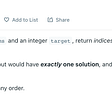# Let’s Explain

`factors: 1, 2, 3, 4, 6, 12`

# Coding up a solution

`function kthFactor(n, k) {  let factors = [];}`
`function kthFactor(n, k) {  let factors = [];for (let i = 1; i <= n; i++) {    if (n % i === 0) factors.push(i);  }}`
`function kthFactor(n, k) {  let factors = [];for (let i = 1; i <= n; i++) {    if (n % i === 0) factors.push(i);    if (factors.length === k) return factors[k - 1];  }  return -1;}`

# Code Walk Through

• We begin looping at i = 1
• Does 12 / 1 have a remainder? Nope, so we push 1 into our factors array.
• Now our factors array is , which is of length 1. Since 1 is not 3, we skip the next conditional and increment i to 2.
• Does 12 / 2 have a remainder? Also no. So we push 2 into our factors array.
• Now our factors array is [1, 2], which is of length 2. Since 2 is not 3, we skip the next conditional and increment i to 3.
• Does 12 / 3 have a remainder? Also no. So we. push 3 into our factors array.
• Now our factors array is [1, 2, 3], which is of length 3. And now 3 = 3, so our next condition evaluates to true. So we return the number in our factors array at k — 1 (remember to account for the whole arrays starting at 0 index, but we started looping at i = 1 in order to return the nth element of a list), which is factors => 3.

--

--

--

## More from Saul Feliz

Always learning.

Love podcasts or audiobooks? Learn on the go with our new app.

## Exploring NestJS — Nest’s module system## Wordpress letsmakeparty3 redirect hack fix## Learning ReactJS while brushing up my Javascript knowledge (& fighting with command line & other…## 20 String Methods in Javascript## Is There an API To Get Precious Metals Rates In Pound Sterling With JSON Format?Always learning.

## Leetcode: Two Sum (JS)## Byte size info: Stack data Structures## LeetCode #994: Rotting Oranges Problem## How to sort an array of object values based on string?